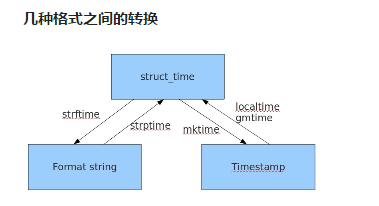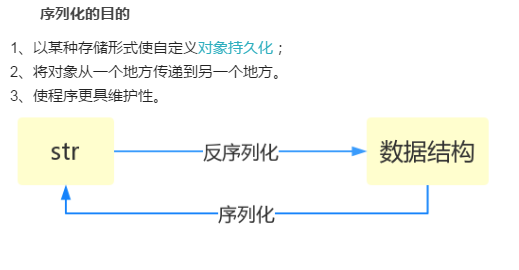# collection、time、os、sys、random、序列化模块

1.具名元组 namedtuple

一般用于扑克洗牌、坐标等场景from collections import namedtuple
point = namedtuple('坐标',['x','y','z'])  # 第二个参数既可以传可迭代对象
# point = namedtuple('坐标','x y z')  # 也可以传字符串 但是字符串之间以空格隔开
p = point(1,2,5)  # 注意元素的个数必须跟namedtuple第二个参数里面的值数量一致
print(p)  # 坐标(x=1, y=2, z=5)
print(p.x)  # 1
print(p.y)  # 2
print(p.z)  # 5
View Code

2、queue 队列 ：先进先出（FIFO ：first in first out）

队列不应该支持任意位置插值，只能在首尾插值

q.put() 往队列中添加值

q.get() 朝队列要值import queue
q = queue.Queue()  # 生成队列对象
q.put('first')  # 往队列中添加值
q.put('second')
q.put('third')

print(q.get())  # 朝队列要值
print(q.get())
print(q.get())
print(q.get())  # 如果队列中的值取完了 程序会在原地等待 直到从队列中拿到值才停止
View Code

deque 双端队列

可以根据索引在任意位置插值

deque除了实现list的append()pop()外，还支持appendleft()popleft()，这样就可以非常高效地往头部添加或删除元素。from collections import deque
q = deque(['a','b','c'])
q.append(1)
q.appendleft(2)

q.insert(0,'哈哈哈')  # 特殊点:双端队列可以根据索引在任意位置插值
print(q.pop())  # 1
print(q.popleft())  # 哈哈哈
print(q.popleft())  # 2
View Code

3、OrderedDict  有序字典、

OrderedDict的Key会按照插入的顺序排列，不是Key本身排序from collections import OrderedDict
order_d = OrderedDict([('a',1),('b',2),('c',3)])  #  有序字典

order_d1 = OrderedDict()
order_d1['x'] = 1
order_d1['y'] = 2
order_d1['z'] = 3
print(order_d1)  # OrderedDict([('x', 1), ('y', 2), ('z', 3)])

order_d1 = dict()
print(order_d1)  # {'x': 1, 'y': 2, 'z': 3}
View Code

4、defauldict

使dict时，如果引用的Key不存在，就会抛出KeyError。如果希望key不存在时，返回一个默认值，就可以用defaultdict

key对应的value无值，默认是空from collections import defaultdict

values = [11, 22, 33,44,55,66,77,88,99,90]
my_dict = defaultdict(list)  # 后续该字典中新建的key对应的value默认就是列表
print(my_dict['aaa'])   #  []   空列表

for value in  values:
if value>66:
my_dict['k1'].append(value)
else:
my_dict['k2'].append(value)
print(my_dict)
# defaultdict(<class 'list'>, {'aaa': [], 'k2': [11, 22, 33, 44, 55, 66], 'k1': [77, 88, 99, 90]})
View Code

可以是列表，int，布尔值，tuplefrom collections import defaultdict

values = [11, 22, 33,44,55,66,77,88,99,90]

my_dict1 = defaultdict(int)
print(my_dict1['xxx'])  # 0
print(my_dict1['yyy'])  # 0

my_dict2 = defaultdict(bool)
print(my_dict2['kkk'])  #  False

my_dict3 = defaultdict(tuple)
print(my_dict3['mmm'])  # ()
View Code

5、Counter

Counter类的目的是用来跟踪值出现的次数。它是一个无序的容器类型，以字典的键值对形式存储，其中元素作为key，其计数作为value。计数值可以是任意的Interger（包括0和负数）

from collections import Counter
s = 'abcdeabcdabcaba'
res = Counter(s)
print(res)  # Counter({'a': 5, 'b': 4, 'c': 3, 'd': 2, 'e': 1})

1、import time

和时间有关系的我们就要用到时间模块。在使用模块之前，应该首先导入这个模块。

三种表现形式

1、时间戳　　time.time()

2、格式化时间（用来展示给人看的）  　　time.strftime()

print(time.strftime('%Y-%m-%d %H:%M:%S'))

print(time.strftime('%Y-%m-%d %X'))    # %X等价于%H:%M:%S

3、结构化时间（元组）用来操作时间 　　 time.localtime()

常用方法%y 两位数的年份表示（00-99）
%Y 四位数的年份表示（000-9999）
%m 月份（01-12）
%d 月内中的一天（0-31）
%H 24小时制小时数（0-23）
%I 12小时制小时数（01-12）
%M 分钟数（00=59）
%S 秒（00-59）
%a 本地简化星期名称
%A 本地完整星期名称
%b 本地简化的月份名称
%B 本地完整的月份名称
%c 本地相应的日期表示和时间表示
%j 年内的一天（001-366）
%p 本地A.M.或P.M.的等价符
%U 一年中的星期数（00-53）星期天为星期的开始
%w 星期（0-6），星期天为星期的开始
%W 一年中的星期数（00-53）星期一为星期的开始
%x 本地相应的日期表示
%X 本地相应的时间表示
%Z 当前时区的名称
%% %号本身
python中时间日期格式化符号：#导入时间模块
>>>import time

#时间戳
>>>time.time()
1500875844.800804

#时间字符串
>>>time.strftime("%Y-%m-%d %X")
'2017-07-24 13:54:37'
>>>time.strftime("%Y-%m-%d %H-%M-%S")
'2017-07-24 13-55-04'

#时间元组:localtime将一个时间戳转换为当前时区的struct_time
time.localtime()
time.struct_time(tm_year=2017, tm_mon=7, tm_mday=24,
tm_hour=13, tm_min=59, tm_sec=37,
tm_wday=0, tm_yday=205, tm_isdst=0)
python时间表现几种格式2、import datetime

datetime.datetime.today() 年月日 时分秒

datetime.date.today() 年月日

　　日期对象 = 日期对象 +/- timedelta对象　　timedelta对象 = 日期对象 +/- 日期对象import datetime
print(datetime.date.today())  # date>>>:年月日
print(datetime.datetime.today())  # datetime>>>:年月日 时分秒
res = datetime.date.today()
res1 = datetime.datetime.today()
print(res.year)
print(res.month)
print(res.day)
print(res.weekday())  # 0-6表示星期  0表示周一

print(res.isoweekday())  # 1-7表示星期 7就是周日
"""
(******)

timedelta对象 = 日期对象 +/- 日期对象
"""
datetime

对时间进行操作current_time = datetime.date.today()  # 日期对象
timetel_t = datetime.timedelta(days=7)  # timedelta对象
res1 = current_time+timetel_t  # 日期对象

print(current_time - timetel_t)  # 2019-07-11

print(res1-current_time)  # 7 days, 0:00:00
View Code

import random

random.randint 　　随机取一个你提供的整数范围内的数字 包含首尾

random.random　　 随机取0-1之间小数

random.choice　　摇号 随机从列表中取一个元素

random.shuffle　　　洗牌

import random

print(random.randint(1,6))  # 随机取一个你提供的整数范围内的数字  包含首尾
print(random.random())  # 随机取0-1之间小数
print(random.choice([1,2,3,4,5,6]))  # 摇号 随机从列表中取一个元素
res = [1,2,3,4,5,6]
random.shuffle(res)  # 洗牌
print(res)

'''
3
0.3075844532784746
2
[4, 3, 6, 1, 2, 5]
''''''

5位数的随机验证码
chr
random.choice

'''
def get_code(n):
code = ''
for i in range(n):
# 先生成随机的大写字母 小写字母 数字
upper_str = chr(random.randint(65,90))
lower_str = chr(random.randint(97,122))
random_int = str(random.randint(0,9))
# 从上面三个中随机选择一个作为随机验证码的某一位
code += random.choice([upper_str,lower_str,random_int])
return code
res = get_code(4)
print(res)

os模块:跟操作系统打交道的模块

1、os.mkdir('文件夹') 　　 自动创建文件夹

2、os.path.exists(r'文件路径')　　 判断文件是否存在

3、os.path.isfile(r'文件路径'） 　　判断文件夹是否存在，存在返回True

4、os.path.getsize（r'文件路径'）　　获取文件大小os.makedirs('dirname1/dirname2')    可生成多层递归目录
os.removedirs('dirname1')    若目录为空，则删除，并递归到上一级目录，如若也为空，则删除，依此类推
os.mkdir('dirname')    生成单级目录；相当于shell中mkdir dirname
os.rmdir('dirname')    删除单级空目录，若目录不为空则无法删除，报错；相当于shell中rmdir dirname
os.listdir('dirname')    列出指定目录下的所有文件和子目录，包括隐藏文件，并以列表方式打印
os.remove()  删除一个文件
os.rename("oldname","newname")  重命名文件/目录
os.stat('path/filename')  获取文件/目录信息

os.system("bash command")  运行shell命令，直接显示
os.popen("bash command).read()  运行shell命令，获取执行结果
os.getcwd() 获取当前工作目录，即当前python脚本工作的目录路径
os.chdir("dirname")  改变当前脚本工作目录；相当于shell下cd

os.path
os.path.abspath(path) 返回path规范化的绝对路径
os.path.split(path) 将path分割成目录和文件名二元组返回
os.path.dirname(path) 返回path的目录。其实就是os.path.split(path)的第一个元素
os.path.basename(path) 返回path最后的文件名。如何path以／或\结尾，那么就会返回空值。即os.path.split(path)的第二个元素
os.path.exists(path)  如果path存在，返回True；如果path不存在，返回False
os.path.isabs(path)  如果path是绝对路径，返回True
os.path.isfile(path)  如果path是一个存在的文件，返回True。否则返回False
os.path.isdir(path)  如果path是一个存在的目录，则返回True。否则返回False
os.path.join(path1[, path2[, ...]])  将多个路径组合后返回，第一个绝对路径之前的参数将被忽略
os.path.getatime(path)  返回path所指向的文件或者目录的最后访问时间
os.path.getmtime(path)  返回path所指向的文件或者目录的最后修改时间
os.path.getsize(path) 返回path的大小
os.path.用法

sys模块:跟python解释器打交道模块import sys
# sys.path.append()  # 将某个路径添加到系统的环境变量中
print(sys.platform)     # 当前系统位数

print(sys.version)  # python解释器的版本

print(sys.argv)  # 命令行启动文件 可以做身份的验证

if len(sys.argv) <= 1:
print('请输入用户名和密码')
else:
username = sys.argv
password = sys.argv
if username == 'jason' and password == '123':
print('欢迎使用')
# 当前这个py文件逻辑代码
else:
print('用户不存在 无法执行当前文件')
sys用法

序列：字符串

序列化：其他数据类型转化成字符串的过程

反序列化:字符串转成其他数据类型ps：写入文件的数据必须是字符串

基于网络传输的数据必须是二进制

import  json

json模块

json格式的字符串 必须是双引号

所有的语言都支持json格式

支持的数据类型很少，字符串，列表，字典，整形，元组（转化成列表），布尔值

1、dumps:序列化 　　将其他数据类型转成json格式的字符串

loads:反序列化 　　将json格式的字符串转换成其他数据类型import json
d = {"name":"jason"}
print(d)  #  {'name': 'jason'}

res = json.dumps(d)
# json格式的字符串 必须是双引号 >>>: '{"name": "jason"}'

print(res,type(res))  # {"name": "jason"} <class 'str'>
res1 = json.loads(res)
print(res1,type(res1))  # {'name': 'jason'} <class 'dict'>
View Code

2、dump   用于文件读写

loadimport josn

d = {"name":"jason"}
with open('userinfo','w',encoding='utf-8') as f:
json.dump(d,f)  # 装字符串并自动写入文件
with open('userinfo','r',encoding='utf-8') as f:
res = json.load(f)
print(res,type(res))

import  pickle

pickle模块

和json用法一样，序列化都是转化成二进制

文件的打开模式必须是b模式

只支持python

python所有的数据类型都支持

******import pickle
d = {'name':'jason'}
res = pickle.dumps(d)  # 将对象直接转成二进制

print(pickle.dumps(d))  #b'\x80\x03}q\x00X\x04\x00\x00\x00nameq\x01X\x05\x00\x00\x0
0jasonq\x02s.'

res1 = pickle.loads(res)
print(res1,type(res1)) # {'name': 'jason'} <class 'dict'>
View Code

sbu：子

process:进程"""
1.用户通过网络连接上了你的这台电脑
2.用户输入相应的命令 基于网络发送给了你这台电脑上某个程序
3.获取用户命令 里面subprocess执行该用户命令
4.将执行结果再基于网络发送给用户

"""
# while True:
#     cmd = input('cmd>>>:').strip()
#     import subprocess
#     obj = subprocess.Popen(cmd,shell=True,stdout=subprocess.PIPE,stderr=subprocess.PIPE)
#     # print(obj)
#     print('正确命令返回的结果stdout',obj.stdout.read().decode('gbk'))
#     print('错误命令返回的提示信息stderr',obj.stderr.read().decode('gbk'))
View Code

posted @ 2019-07-18 21:07 imcate 阅读(...) 评论(...) 编辑 收藏